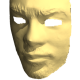# When does cvEstimateRigidTransform recognise 2D point sets instead of images

Firstly I can not find the C documentation of this function, only for C++: http://docs.opencv.org/modules/video/doc/motion_analysis_and_object_tracking.html#Mat%20estimateRigidTransform%28InputArray%20src,%20InputArray%20dst,%20bool%20fullAffine%29

This is the C prototype I could find:

CVAPI(int)  cvEstimateRigidTransform( const CvArr* A, const CvArr* B,
CvMat* M, int full_affine );


It describes that the 2D point set should be given as vector or Mat. Since there are no vectors in C I have to use CvMat instead. If there is another way to represent a point set which is conform with the CvArr* metatype please tell me.

What I currently try is storing each Point of interest in CvMat's as a value > 0.

CvMat* new_frame = cvCreateMat(height, width, CV_8UC1);
CvMat* old_frame = cvCreateMat(height, width, CV_8UC1);


I do not know if this is correct! The relevant points or rather edges of an object are extracted using the Sobel Operator on a gray scaled image. The actual transformation from the old to the new Frame is then computed:

trans = cvCreateMat(2, 3, CV_64FC1);
cvEstimateRigidTransform(new_frame, old_frame, trans, 0);


Now, it could be, this function takes new_frame and old_frame as point sets, which would be correct or as plain Images, where it would use some further magic on it, i do not wish for. How do I avoid the additional magic?

Concluding what is relevant:

• Is there another way to define point set for use with CvArr*
• If not, how do I define a 2D point set in the CvMat's so it explicitly recognises them as such.
• Are the types for the Input Mats CV_MAT_8UC1 and output mat CV_MAT_64FC1 correct?

Also a side note: When i have the type of the transformation matrix a CV_MAT_64FC1 the result is definitly wrong. If I use CV_MAT_32FC1 instead the result seems to be somewhat correct. However, everywhere i looked the type of the matrix was CV_MAT_64FC1, so this confuses me.

Whoever replies: Thanks :)

edit retag close merge delete

Sort by » oldest newest most votedIt is a bit confusing at first, but this kind of input gives you some freedom about the input type. You do not pass a image as input, rather a array of elements which represents your 2D point set.

It can be:

1. std::vector<cv::Point2f>
2. std::vector<cv::Vec2f>
3. cv::Mat with N lines and 1 column, with type CV_32FC2, each element consists in 2 floating point members) , such that can be represented by cv::Vec2f

Particularly, I use mostly the first option to represent the point set. If you don't know what is std::vector container, I suggest you give a look in some tutorials of STL containers here. Understand those containers is helpful to you understand certain OpenCV functions and structures.

Edit: Sorry, now I noticed that you are using C interface. I don't recommend it very much, however, if you do not have choice about this, use the 3rd option.

more

Yeah unfortunatly I am restricted to a C framework. Your 3rd option for representing the points most likely is the part I misunderstood. Where did you find this information, couldn't find it in the docu. But thanks, i reimplement the stuff and mark it as answered after the test :).

Ok reimplemented it. It seems it only accepts point sets which have exactly the same amount of points. Throws following: "Both input images must have the same size in function cvEstimateRigidTransform". When u extract the edge features in an Image you will always have different amount of points in consecutive frames. So this function can't handle the most occuring case. Maybe it is not designed for that purpose(tracking in consecutive frames)? I have no clue.

In order to compute a transform you have to know the correspondence of points in each image. In fact, you should detect the points in a reference frame, compute the correspondence in the following frame by an approach such as optical-flow or feature matching. When you have a set of points that were successfully matched, you compute the desired transform. The algorithm of choice to you compute this correspondences depends a lot of the nature of the application. Optical-flow works well when you have frames which do not change very much and you need fast computation. Feature-matching is more suited to stitching or other tasks where the frame varies a lot and you need invariance regarding rotation, translation or scale. This function wont help you with tracking directly.

I thought it would find the transformation via computing the transformation with the lowest cost and therefore creating the correspondence(kinda like a KLT tracker). Guess i got the order wrong. It kinda works with raster images though, although they have a different amount of points. The extracted edge features should complement the overall tracking(with a static camera) combined with depth data to resolve occlusion of >2 objects better on same depth levels, I use it next to colour histogramms. Thanks for your help again, i guess this matter here is done.

Official site

GitHub

Wiki

Documentation